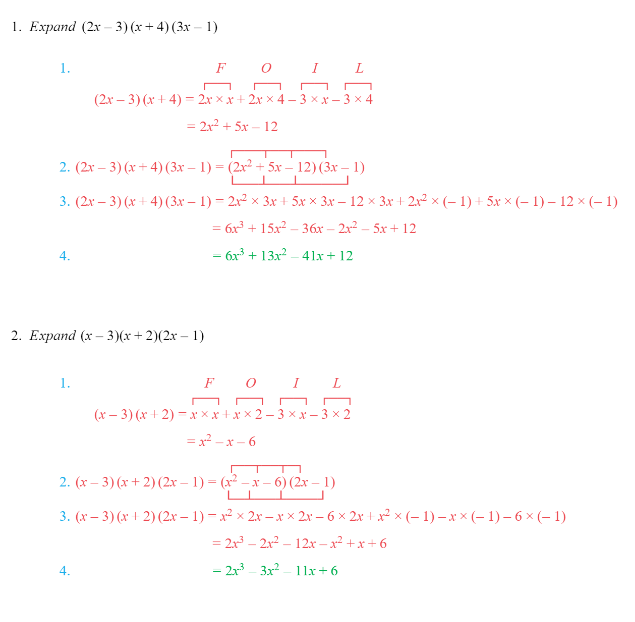# Edexcel IGCSE Maths 复习笔记 2.3.3 Expanding Three Brackets

Edexcel IGCSE Maths 复习笔记 2.3.3 Expanding Three Brackets

#### Expanding three brackets

• We’re still following the basic principle of expanding here – get rid of the brackets by multiplying out
• We just need a good method to make sure everything gets multiplied by everything else in the correct way

#### Beware of minus signs

• Remember the basic rules:

“ − × − = + ”“ − × + = − ”

#### How to multiply out three brackets

• Multiply out one pair of brackets using FOIL (as for two brackets)
• If the new bracket has two terms use FOIL (again!)
• Otherwise link each term in the smaller bracket to each term in the larger bracket (that’s six links in total)
• Write down the six multiplications
• Careful here – you have to be sure to include any minus signs!
• Simplify by collecting like terms (if there are any)

#### Worked Example